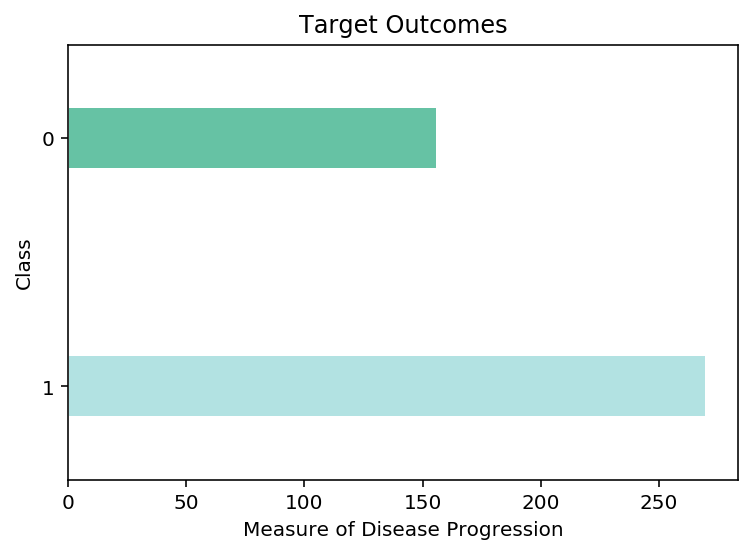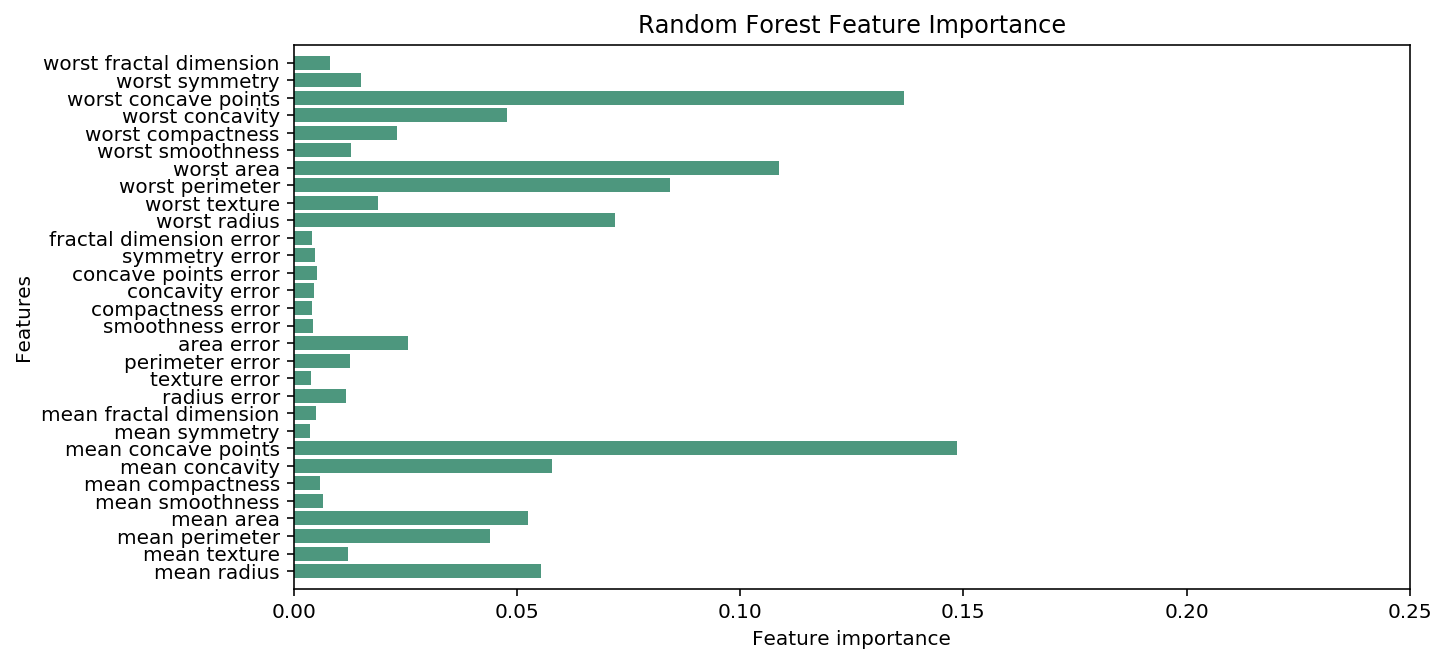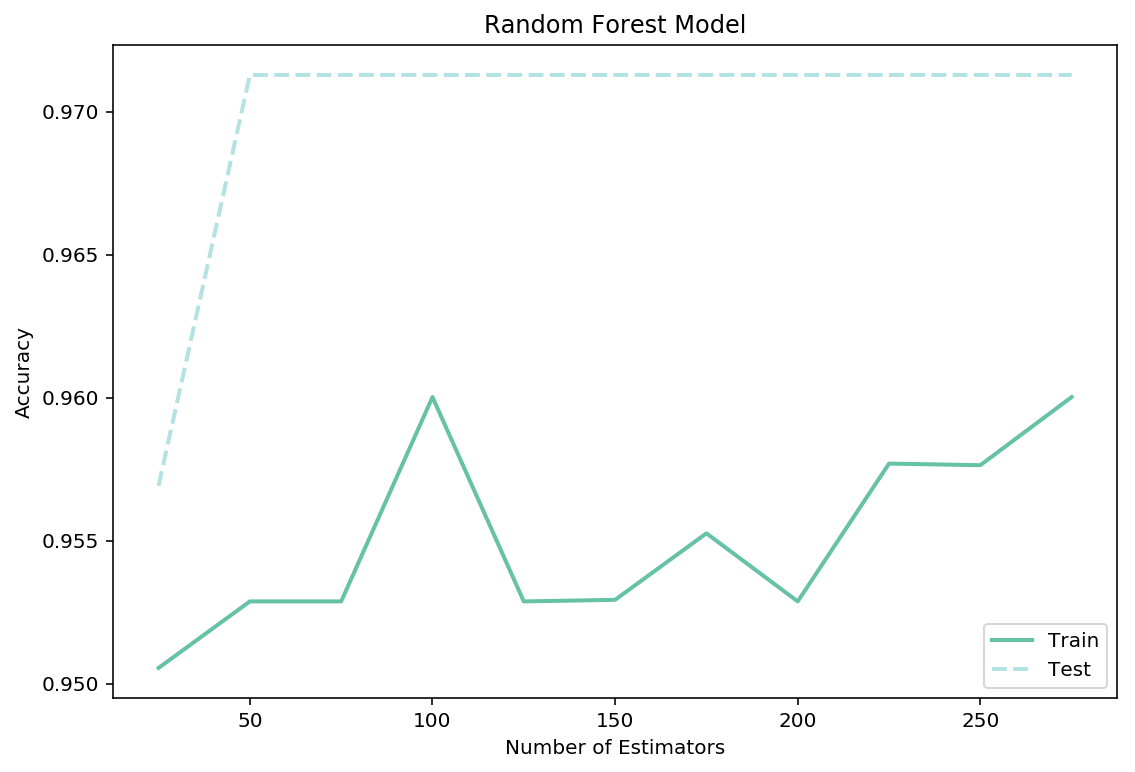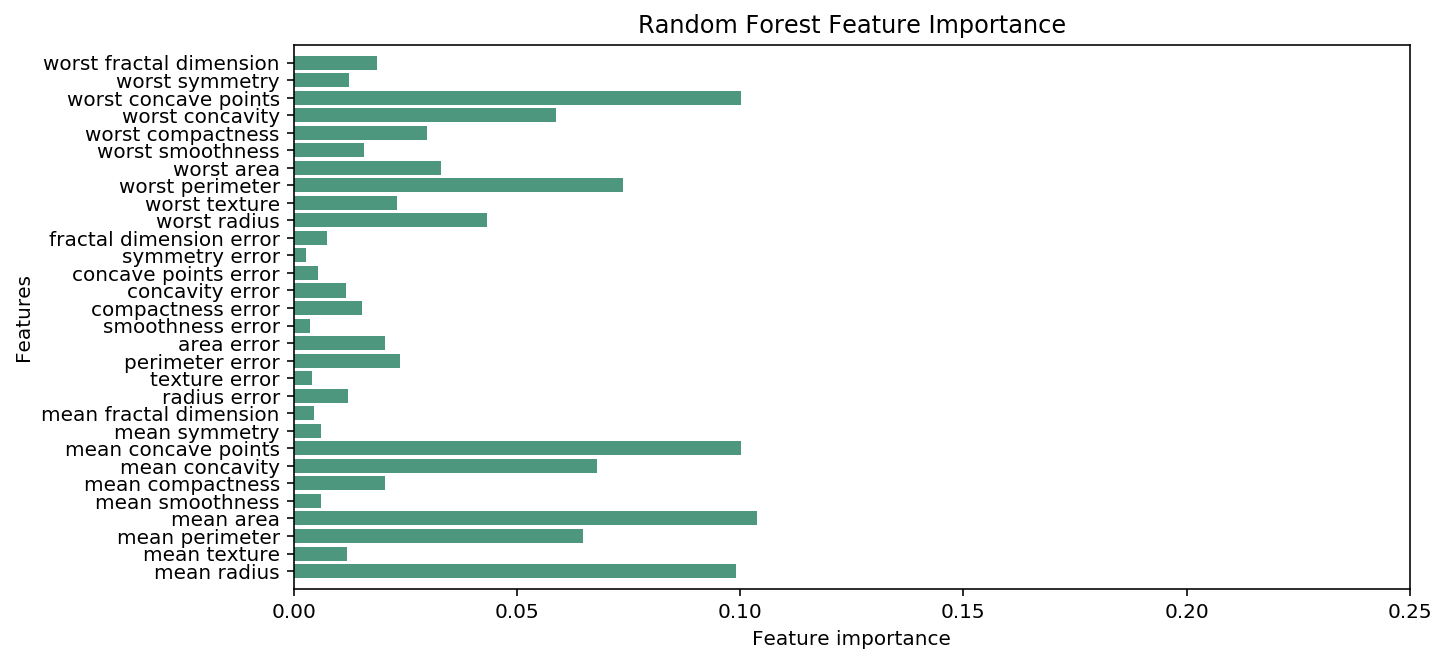14 Sep

## Random Forest Classifier

The Random Forest Classifications algorithim use a ensemble of Decision Trees whent training its model. Each decision tree in the ensemble is trained on a random subset of the features. At the same time each decicision tree in the ensemble is meant to overfit the data in some manner. After each decisions tree is trained the ensemble averages the results between each all the trees to gets it's model.

In this notebook, I will be looking at the famous breastcancer dataset. This dataset is a multi-class classification problem, where I need to predict the correct target for each observation from a range of possible classes. We will attempt to predict the proper target class using this model, given the feature of each type of class, I often reuse this dataset between my tree-based notebooks. Using the same dataset makes it very easy to compare and contrast the performance of different tree-based models, and keep the trees a reasonable size.

Dataset: Breast Cancer

### Import Preliminaries¶

In :
%matplotlib inline
%config InlineBackend.figure_format='retina'

# Import modules
import matplotlib.pyplot as plt
import matplotlib as mpl
import numpy as np
import pandas as pd
import seaborn
import warnings

from sklearn.model_selection import train_test_split, cross_val_score, GridSearchCV
from sklearn.ensemble import RandomForestClassifier

# Set pandas options
pd.set_option('max_columns',1000)
pd.set_option('max_rows',30)
pd.set_option('display.float_format', lambda x: '%.3f' % x)

# Set plotting options
mpl.rcParams['figure.figsize'] = (9.0, 3.0)

# Set warning options
warnings.filterwarnings('ignore');


### Import Data¶

In :
# Import Breast Cancer data
X, y = breast_cancer.data, breast_cancer.target

# Conduct a train-test split on the data
train_x, test_x, train_y, test_y = train_test_split(X,y)

# View the training dataframe

Out:
mean radius mean texture mean perimeter mean area mean smoothness mean compactness mean concavity mean concave points mean symmetry mean fractal dimension radius error texture error perimeter error area error smoothness error compactness error concavity error concave points error symmetry error fractal dimension error worst radius worst texture worst perimeter worst area worst smoothness worst compactness worst concavity worst concave points worst symmetry worst fractal dimension
0 11.430 17.310 73.660 398.000 0.109 0.095 0.020 0.019 0.165 0.066 0.284 1.908 1.937 21.380 0.007 0.017 0.012 0.010 0.023 0.004 12.780 26.760 82.660 503.000 0.141 0.179 0.077 0.064 0.258 0.081
1 13.880 16.160 88.370 596.600 0.070 0.048 0.020 0.009 0.161 0.055 0.254 0.622 1.709 23.120 0.004 0.014 0.020 0.007 0.016 0.002 15.510 19.970 99.660 745.300 0.085 0.123 0.109 0.045 0.254 0.066
2 14.410 19.730 96.030 651.000 0.088 0.168 0.136 0.066 0.171 0.072 0.881 1.770 4.360 77.110 0.008 0.106 0.100 0.028 0.041 0.023 15.770 22.130 101.700 767.300 0.100 0.247 0.222 0.102 0.227 0.088
3 12.220 20.040 79.470 453.100 0.110 0.115 0.082 0.022 0.212 0.069 0.181 0.796 0.986 12.580 0.006 0.022 0.040 0.010 0.013 0.004 13.160 24.170 85.130 515.300 0.140 0.232 0.353 0.081 0.271 0.088
4 13.400 20.520 88.640 556.700 0.111 0.147 0.144 0.082 0.212 0.073 0.391 0.931 3.093 33.670 0.005 0.023 0.035 0.013 0.017 0.004 16.410 29.660 113.300 844.400 0.157 0.386 0.511 0.205 0.358 0.111

### Data Overview¶

In :
# Plot a barplot of the target clasees
pd.Series(train_y).value_counts().plot.barh(grid=False, color=['#B2E2E2','#66C2A4'], width=0.25,edgecolor='w')
plt.title('Target Outcomes')
plt.ylabel('Class')
plt.xlabel('Measure of Disease Progression');### Fit the Model¶

In :
# Fit the intial model
rf_model = RandomForestClassifier(n_estimators=100)
rf_model.fit(train_x, train_y);


### Model Evaluation¶

##### Cross Validation Score¶
In :
# View the cross validation score of the intial model
scores = cross_val_score(rf_model, train_x, train_y, cv=10,
scoring='accuracy')
print(f'Cross Validation Score: {scores.mean():.5f}')

Cross Validation Score: 0.94817

##### Confustion Matrix¶
In :
# Training Confusion Matrix
from sklearn.metrics import confusion_matrix
cmatrix = pd.DataFrame(confusion_matrix(train_y, rf_model.predict(train_x)))
cmatrix.index.name = 'class'

cmatrix['result'] = 'actual'
cmatrix.set_index('result', append=True, inplace=True)
cmatrix = cmatrix.reorder_levels(['result', 'class'])
cmatrix = cmatrix.stack()
cmatrix = pd.DataFrame(cmatrix)
cmatrix.columns = ['prediction']
cmatrix.unstack()

Out:
prediction
0 1
result class
actual 0 156 0
1 0 270
##### Feature Importance¶
In :
# Plot Tree's Feature Importance
plt.figure(figsize=(10,5))
n_features = breast_cancer.data.shape
plt.barh(range(n_features), rf_model.feature_importances_, align='center', color='#4D977E')
plt.yticks(np.arange(n_features), breast_cancer.feature_names)
plt.title('Random Forest Feature Importance')
plt.xlabel("Feature importance")
plt.ylabel("Features")
plt.ylim(-1, n_features)
plt.xlim(0,0.25);##### Parameter Tuning¶
In :
# Define paraameter range and score lists
n_estimators_range = np.arange(0,300, 25)[1:]
train_score = []
test_score = []

# Train a knn_model for every neighbour value in our list
for i in n_estimators_range:
rf_model=RandomForestClassifier(n_estimators = i).fit(train_x,train_y)
train_score.append(cross_val_score(rf_model, train_x, train_y, cv=10, scoring='accuracy').mean())
test_score.append(cross_val_score(rf_model, test_x, test_y, cv=10, scoring='accuracy').mean())

# Plot our results
mpl.rcParams['figure.figsize'] = (9.0, 6.0)
plt.plot(n_estimators_range,train_score,label="Train",linewidth=2, color='#66C2A4')
plt.plot(n_estimators_range,test_score,label="Test", linewidth=2,linestyle='--', color='#B2E2E2')
plt.legend()
plt.title('Random Forest Model')
plt.xlabel('Number of Estimators')
plt.ylabel('Accuracy');##### Grid Searching Turnning¶
In :
# Set up parameter grid
grid = {'n_estimators':np.arange(0,100, 25)[1:],
'max_depth':list(range(2,30,4)),
'max_features': list(range(2,30,2)),
'max_leaf_nodes':[5,10,25,50,75,100]}

# Conduct gird search
grid_search = GridSearchCV(estimator=rf_model, param_grid=grid,
scoring='accuracy', n_jobs=-1, refit=True, cv=10,
return_train_score=True)
# Fit model
grid_search.fit(train_x,train_y);

# Print out the parameter for the best score
print('Accuracy of best parameters: %.5f'%grid_search.best_score_)
print('Best parameters: %s' %grid_search.best_params_)

Accuracy of best parameters: 0.96714
Best parameters: {'max_depth': 22, 'max_features': 2, 'max_leaf_nodes': 100, 'n_estimators': 25}


### Final Model¶

In :
# Fit the final model
rf_model = RandomForestClassifier(max_depth=6, max_features=4, max_leaf_nodes=50, n_estimators=50)
rf_model.fit(train_x, train_y)

# View the cross validation score of the intial model
scores = cross_val_score(rf_model, train_x, train_y, cv=10,
scoring='accuracy')
print(f'Cross Validation Score: {scores.mean():.5f}')

Cross Validation Score: 0.95770

##### Confustion Matrix¶
In :
# Training confusion matrix
from sklearn.metrics import confusion_matrix
cmatrix = pd.DataFrame(confusion_matrix(train_y, rf_model.predict(train_x)))
cmatrix.index.name = 'class'

cmatrix['result'] = 'actual'
cmatrix.set_index('result', append=True, inplace=True)
cmatrix = cmatrix.reorder_levels(['result', 'class'])
cmatrix = cmatrix.stack()
cmatrix = pd.DataFrame(cmatrix)
cmatrix.columns = ['prediction']
cmatrix.unstack()

Out:
prediction
0 1
result class
actual 0 155 1
1 0 270
##### Feature Importance¶
In :
# Plot ensembles's feature importance
plt.figure(figsize=(10,5))
n_features = breast_cancer.data.shape
plt.barh(range(n_features), rf_model.feature_importances_, align='center', color='#4D977E')
plt.yticks(np.arange(n_features), breast_cancer.feature_names)
plt.title('Random Forest Feature Importance')
plt.xlabel("Feature importance")
plt.ylabel("Features")
plt.ylim(-1, n_features);
plt.xlim(0,0.25)

Out:
(0, 0.25)### Predict Results¶

In :
# Predict the results from our test data

Out:
0    1
1    1
2    0
3    0
4    0
5    1
6    0
dtype: int64

### General Notes¶

-- Feature importance is calculated by aggregating the results across decision trees
-- Random Forests are decisions tree with all the upside and fewer downsides
-- Build has many decision trees has you have time and memory for
-- Adding max features and max leaf nodes to your decision trees might sometimes improve performance
-- Require little to no preprocessing

Author: Kavi Sekhon